Courses

# The Force Method of Analysis: Frames - 2 Civil Engineering (CE) Notes | EduRev

## Civil Engineering (CE) : The Force Method of Analysis: Frames - 2 Civil Engineering (CE) Notes | EduRev

The document The Force Method of Analysis: Frames - 2 Civil Engineering (CE) Notes | EduRev is a part of the Civil Engineering (CE) Course Structural Analysis.
All you need of Civil Engineering (CE) at this link: Civil Engineering (CE)

Support settlements

As discussed in the case of statically indeterminate beams, the reactions are induced in the case of indeterminate frame due to yielding of supports even when there are no external loads acting on it. The yielding of supports may be either linear displacements or rotations of supports (only in the case of fixed supports) .The compatibility condition is that the total displacement of the determinate frame (primary structure) due to external loading and that due to redundant reaction at a given support must be equal to the predicted amount of yielding at that support. If the support is unyielding then it must be equal to zero.

Example 11.3
A rigid frame ABC is loaded as shown in the Fig 11.3a, Compute the reactions if the support D settles by 10 mm. vertically downwards. Assume EI to be constant for all members. Assume E = 200 GPa and I = 10-4 m2.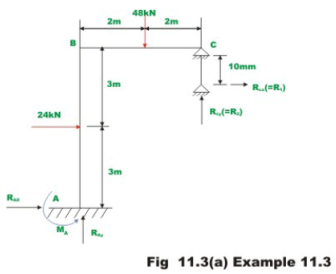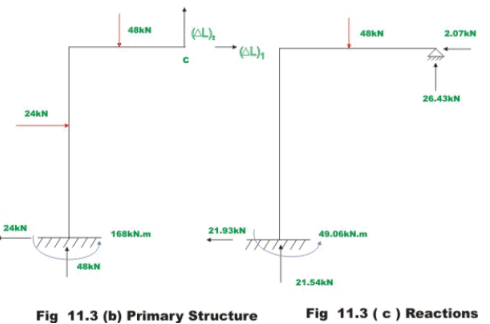This problem is similar to the previous example except for the support settlement .Hence only change will be in the compatibility equations. The released structure is as shown in Fig.11.3b .The deflections (ΔL)1 and (ΔL) at C in the primary structure due to external loading has already been computed in the previous example. Hence,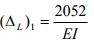(1)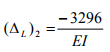(2)

Therefore,

L)= 1026.0 m
L) = − 1635.0 m

The flexibility coefficients are,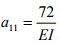(3)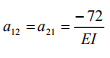(4)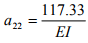(5)

Now, the compatibility equations may be written as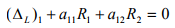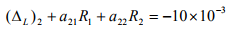(6)

Solving which,

R1 = -2.072 kN (towards left)
R2 = +26.4 kN (upwards)                  (7)

The reactions are shown in Fig.11.3c.

Example 11.4
Compute the reactions of the rigid frame shown in Fig.11.4a and draw bending moment diagram .Also sketch the deformed shape of the frame. Assume EI to be constant for all members.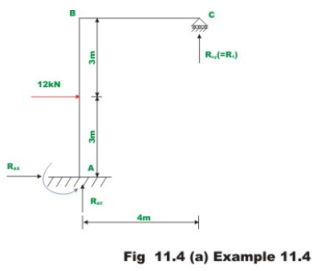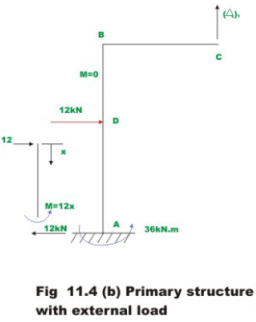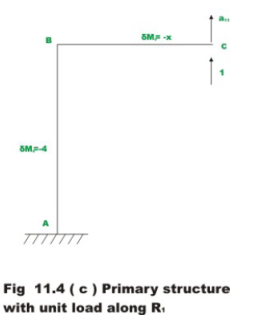Select vertical reaction at C, R1 as the redundant .Releasing constraint against R1 redundant, the primary structure is obtained. It is shown in Fig.11.4b.

The deflection (ΔL)1 in the primary structure due to external loading can be calculated from unit load method.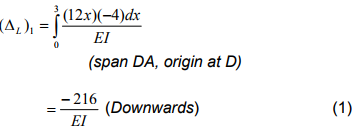Now, compute the flexibility coefficient,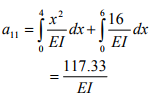(2)

The compatibility condition at support C is that the displacement at C in the primary structure due to external loading plus the displacement at C due to redundant must vanish. Thus,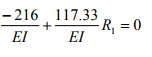(3)

Solving,

R1 = 1.84 kN                                                    (4)

The remaining reactions are calculated from static equilibrium equations. They are shown in Fig.11.4d along with the bending moment diagram.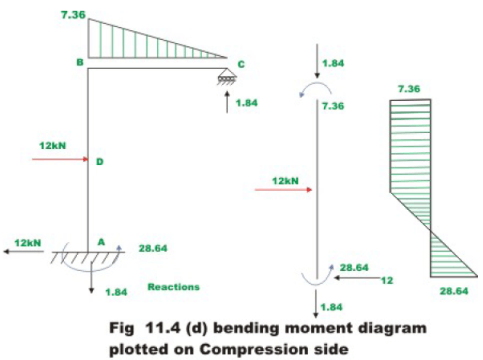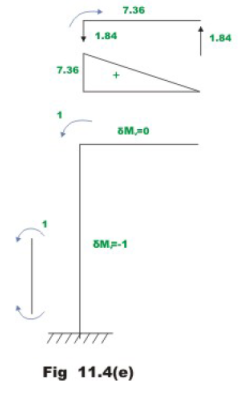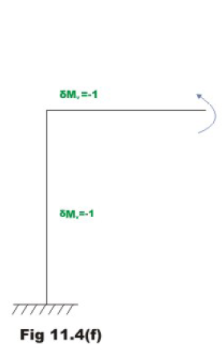To sketch the deformed shape/elastic curve of the frame, it is required to compute rotations of joints B and C and horizontal displacement of C . These joint rotations and displacements can also be calculated from the principle of superposition .The joint rotations are taken to be positive when clockwise. Towards this end first calculate joint rotations at B(θBL) and C(θCL) and horizontal displacement at C in the released structure (refer to Fig.11.4b).This can be evaluated by unit load method.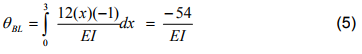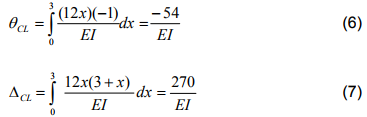Next, calculate the joint rotations and displacements when unit value of redundant is applied (Fig.11.4c). Let the joint rotations and displacements be θBRCR and ΔCR.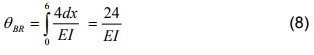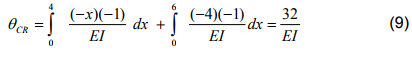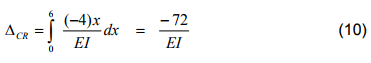Now using the principle of superposition, the actual rotations and displacements at the joints may be obtained.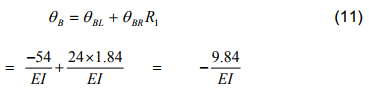(Clockwise rotation)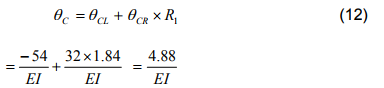(Counterclockwise rotation)

ΔC = ΔCL + ΔCR R1                            (13)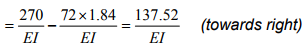The qualitative elastic curve is shown in Fig. 11.4h.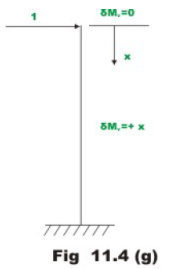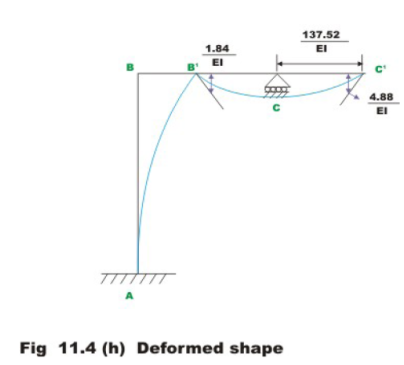Summary

In this lesson, the statically indeterminate plane frames are analysed by force method. For the purpose of illustrations only bending deformations of the frame are considered as the axial deformations are very small. The problem of yielding of supports in the case of plane frames is also discussed. The procedure to draw qualitative elastic curve of the frame is illustrated with the help of typical example. The bending moment and shear force diagrams are also drawn for the case of plane frame.

Offer running on EduRev: Apply code STAYHOME200 to get INR 200 off on our premium plan EduRev Infinity!

## Structural Analysis

30 videos|122 docs|28 tests

,

,

,

,

,

,

,

,

,

,

,

,

,

,

,

,

,

,

,

,

,

;